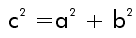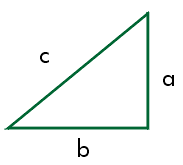The Pythagorean Theorem states that the sum of the squares of the legs of a right triangle is equal to the square of the hypotenuse.Pythagoras (c. 580-500 B.C.) was a philosopher and mathematician. He formed a secret order of mathematicians known as the Pythagorean brotherhood. He is credited for many discoveries in mathematics and geometry. The secretiveness of the brotherhood has made it impossible to determine whether the discoveries were his or one of the other members. His most well known discovery is the Pythagorean Theorem.

Work with the Pythagorean Theorem